# NCERT Exemplar Solutions for Class 11 Maths Chapter 5 Complex Numbers and Quadratic Equations

NCERT Exemplar Solutions for Class 11 Maths Chapter 5 Complex Numbers and Quadratic Equations are considered to be very useful when you are preparing for CBSE Class 11 Maths exams. Here, we bring to you detailed solutions to exercises of the NCERT Exemplar Class 11 Maths Chapter 5. Subject matter experts who created the solutions have compiled these questions for you to revise Chapter 5 of the NCERT Exemplar Textbook. These questions have been devised as per the NCERT syllabus and guidelines. We provide you with accurate solutions to all questions that are covered in the NCERT Exemplar books. These NCERT Exemplar Solutions are based on the latest syllabus. You will get enough practice solving these exercises, and it will also help you to score high marks.

This chapter defines the concept of complex numbers and quadratic equations. A complex number can be defined as a real number, a pure imaginary number or even the sum of both numbers. The real number can be represented as ‘a’, and the imaginary number can be represented as ‘bi’. Quadratic equations are related to imaginary numbers and complex numbers only when the value of the quadratic formula is negative. In Chapter 5 of NCERT Exemplar Solutions for Class 11 Maths, students will learn and solve exemplar problems based on

• Imaginary numbers
• Integral powers of i
• Complex numbers
• Conjugate of a complex number
• Modulus of a complex number
• Properties of modulus of a complex number
• Develop the algebra of complex numbers
• Addition, difference, multiplication and division of two complex numbers
• Power of imaginary number i
• The square roots of a negative real number
• Argand plane and polar representation of the complex number

## Download the PDF of NCERT Exemplar Class 10 Maths Solutions Chapter 5 – Complex Numbers and Quadratic Equations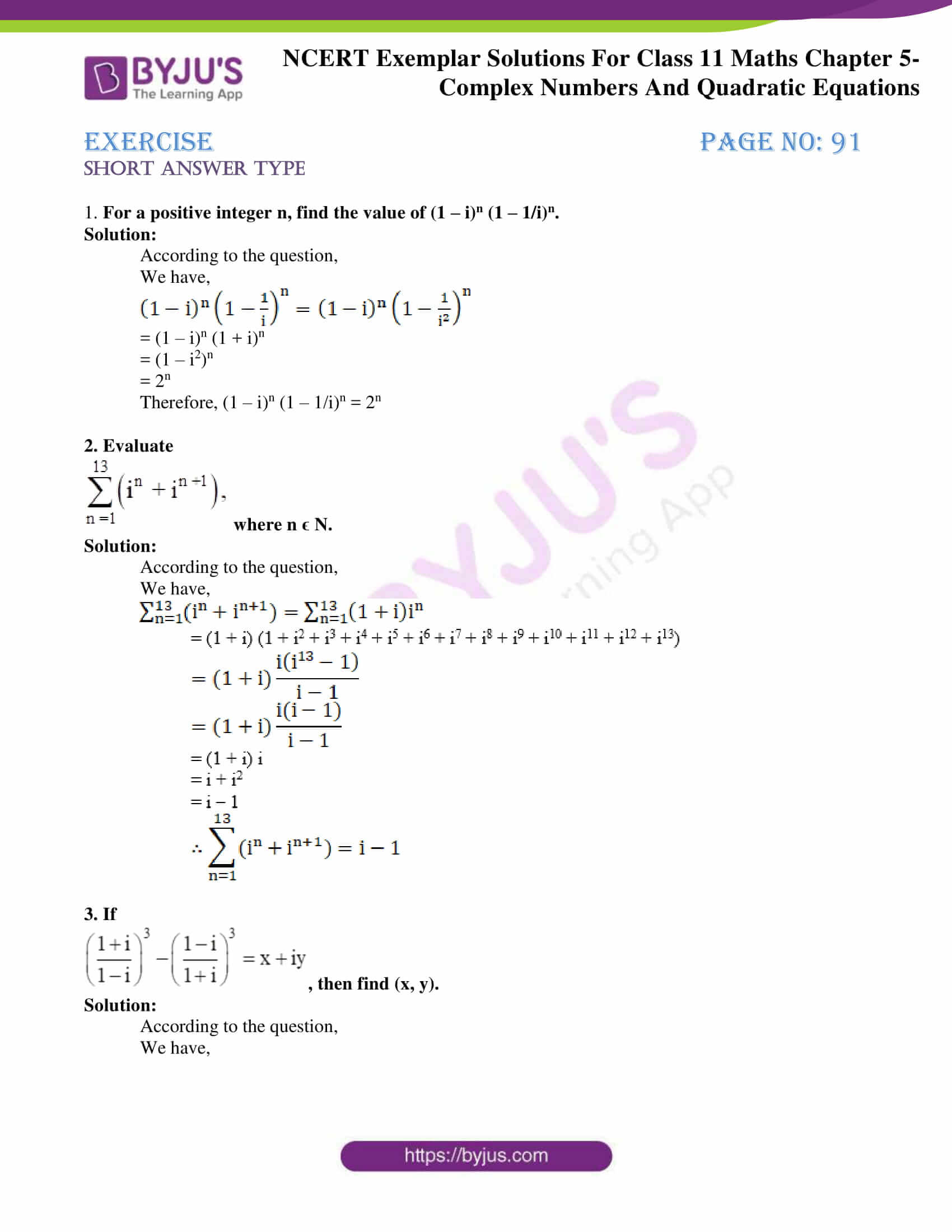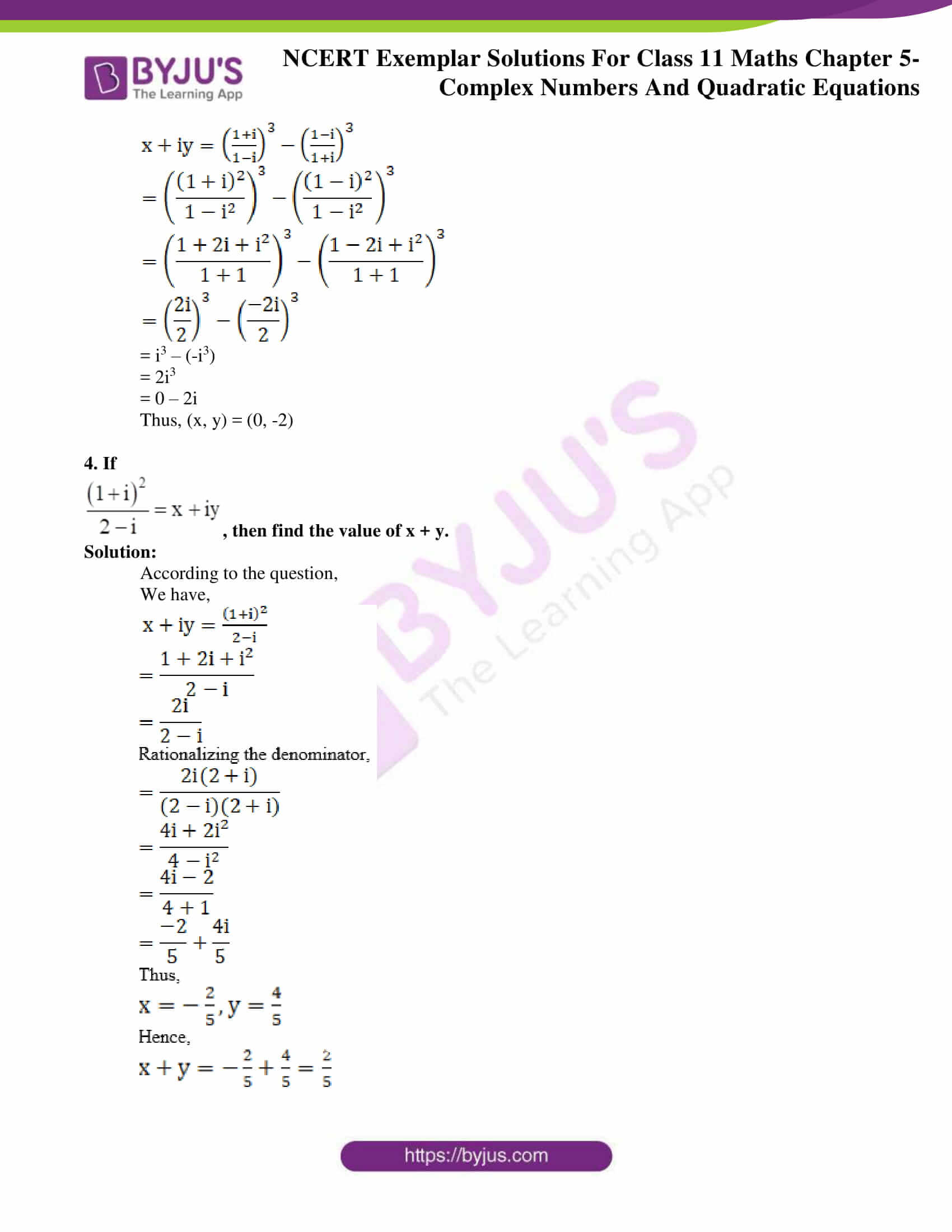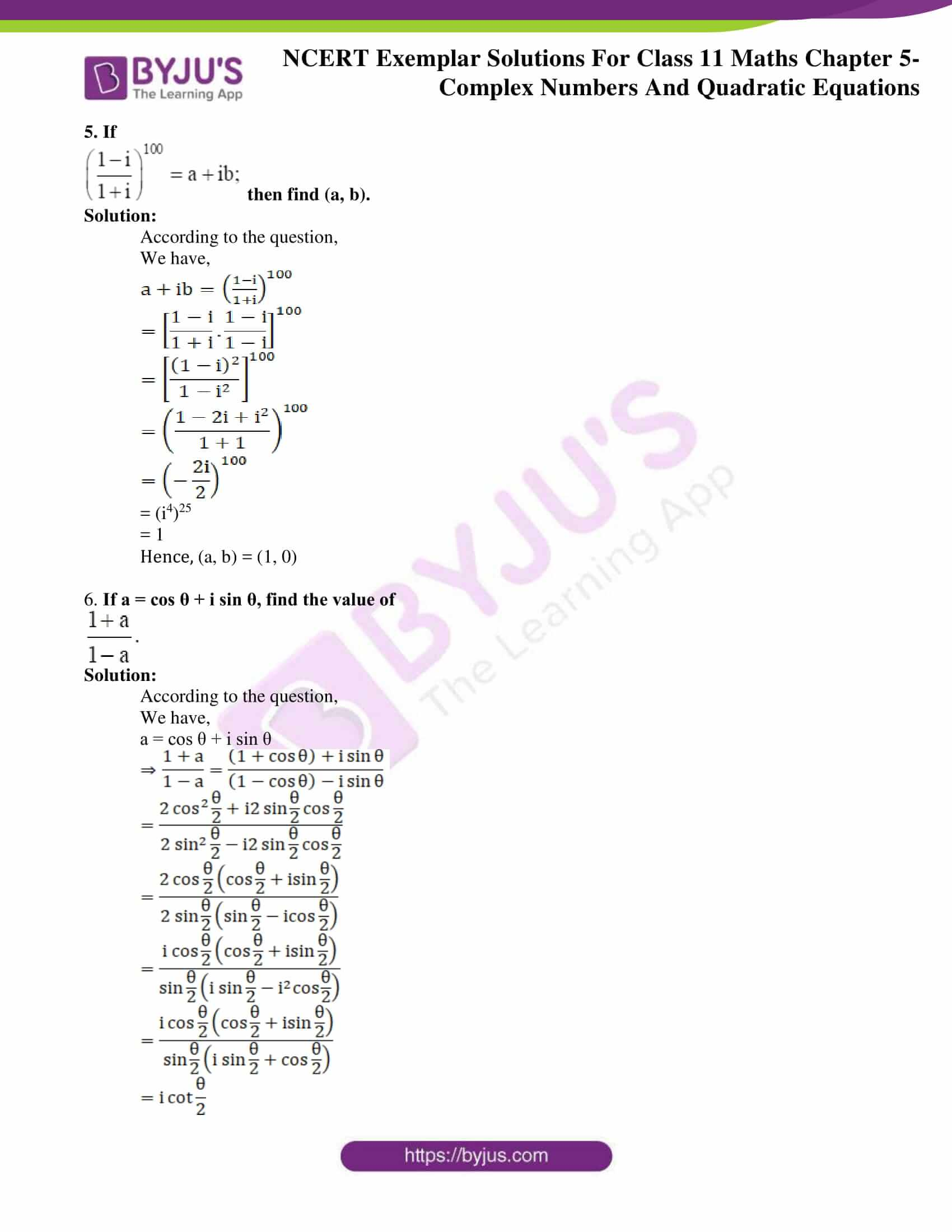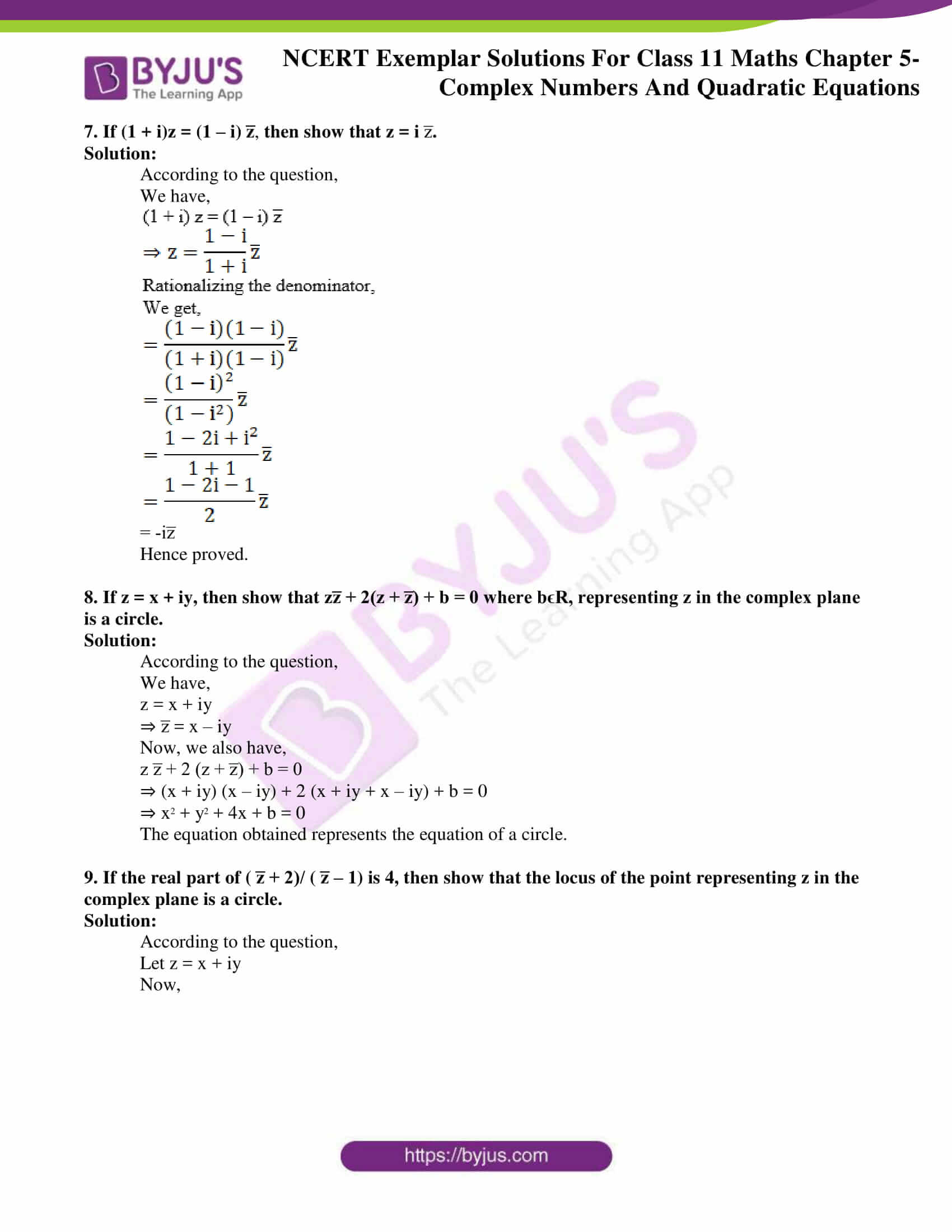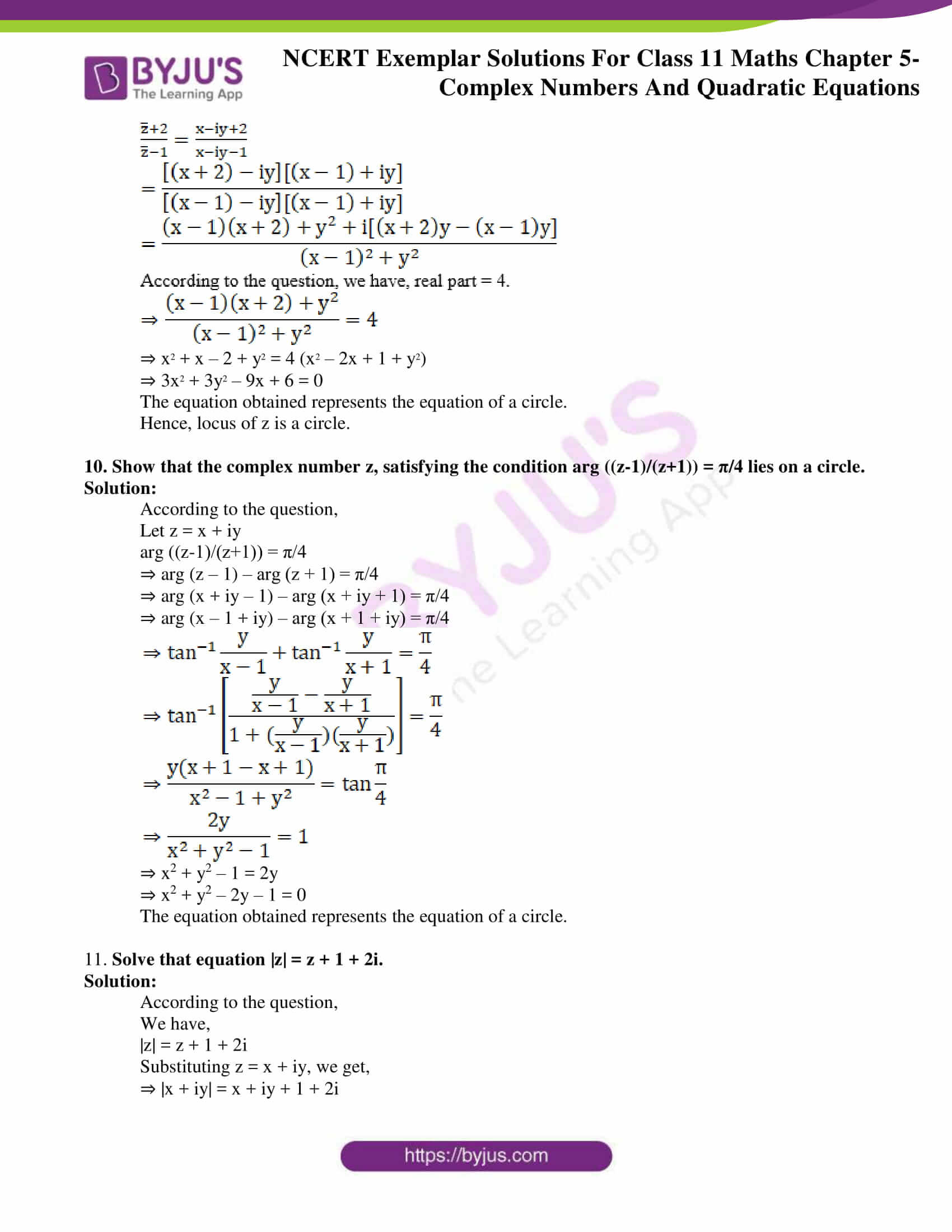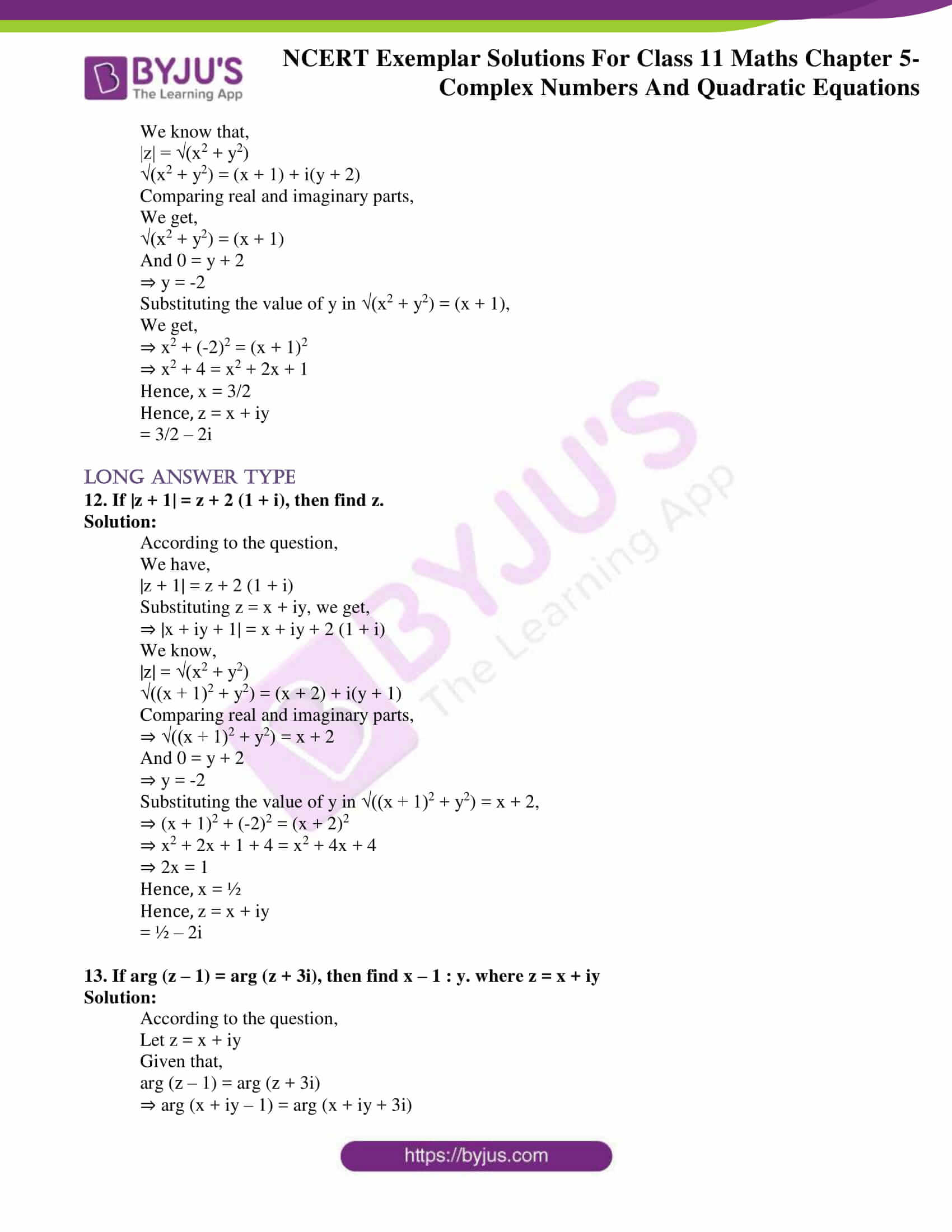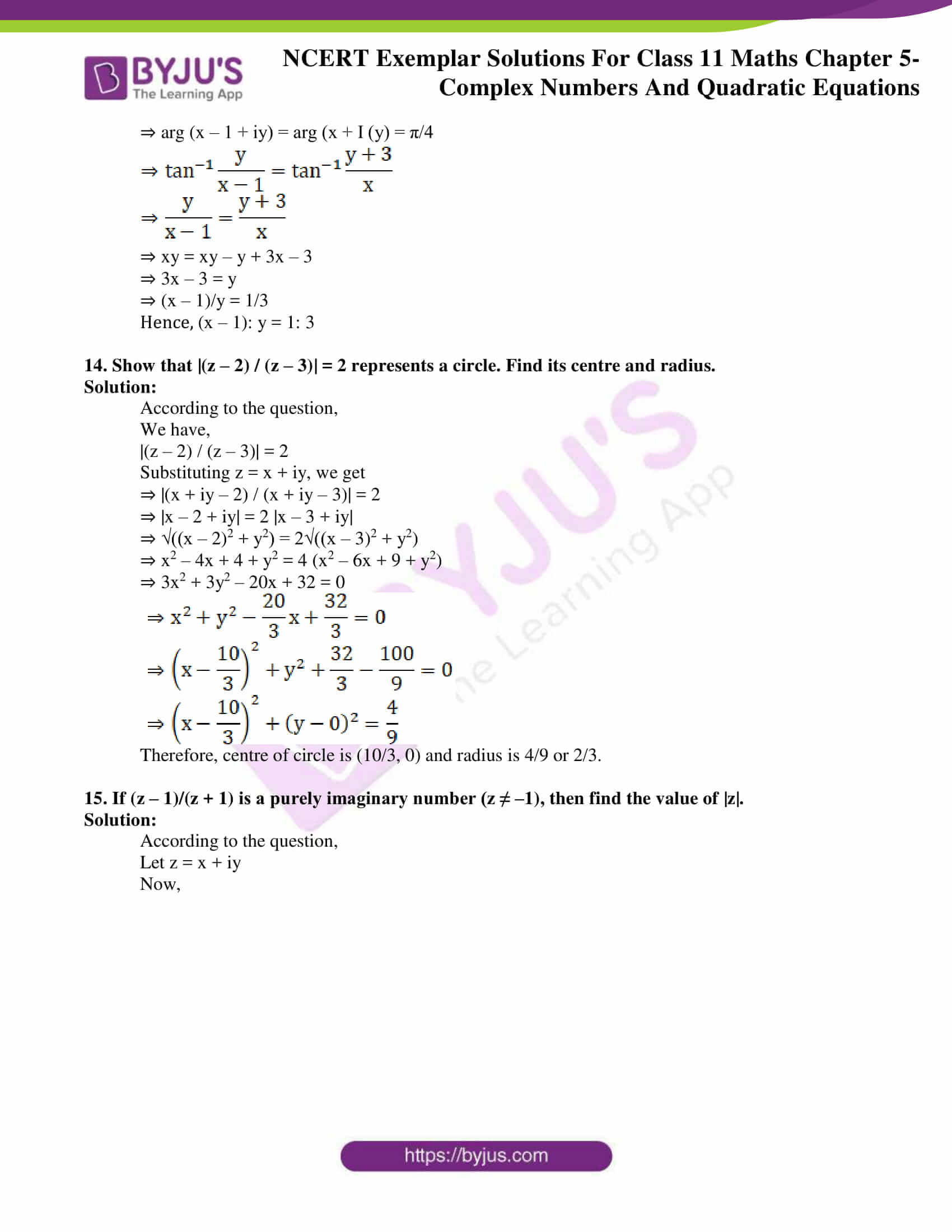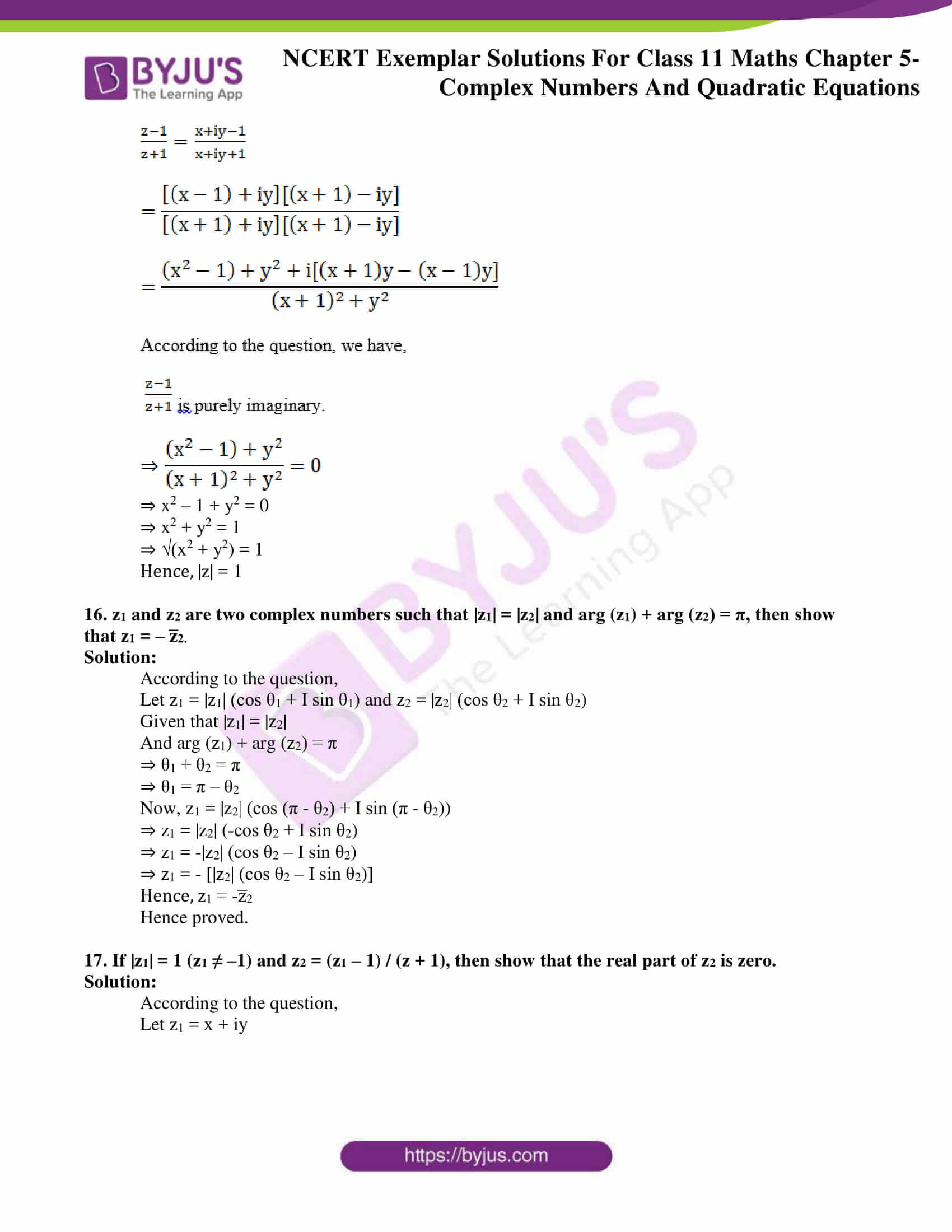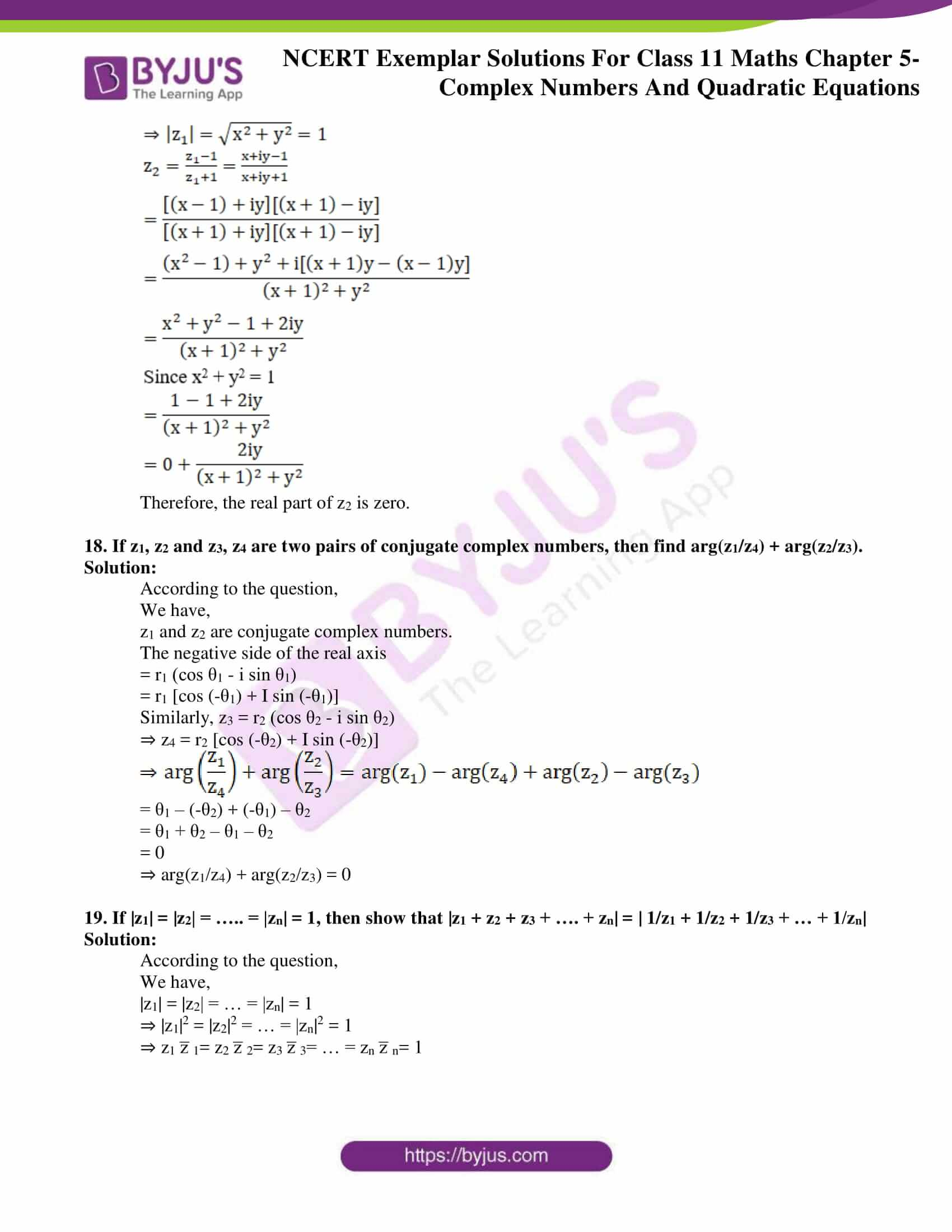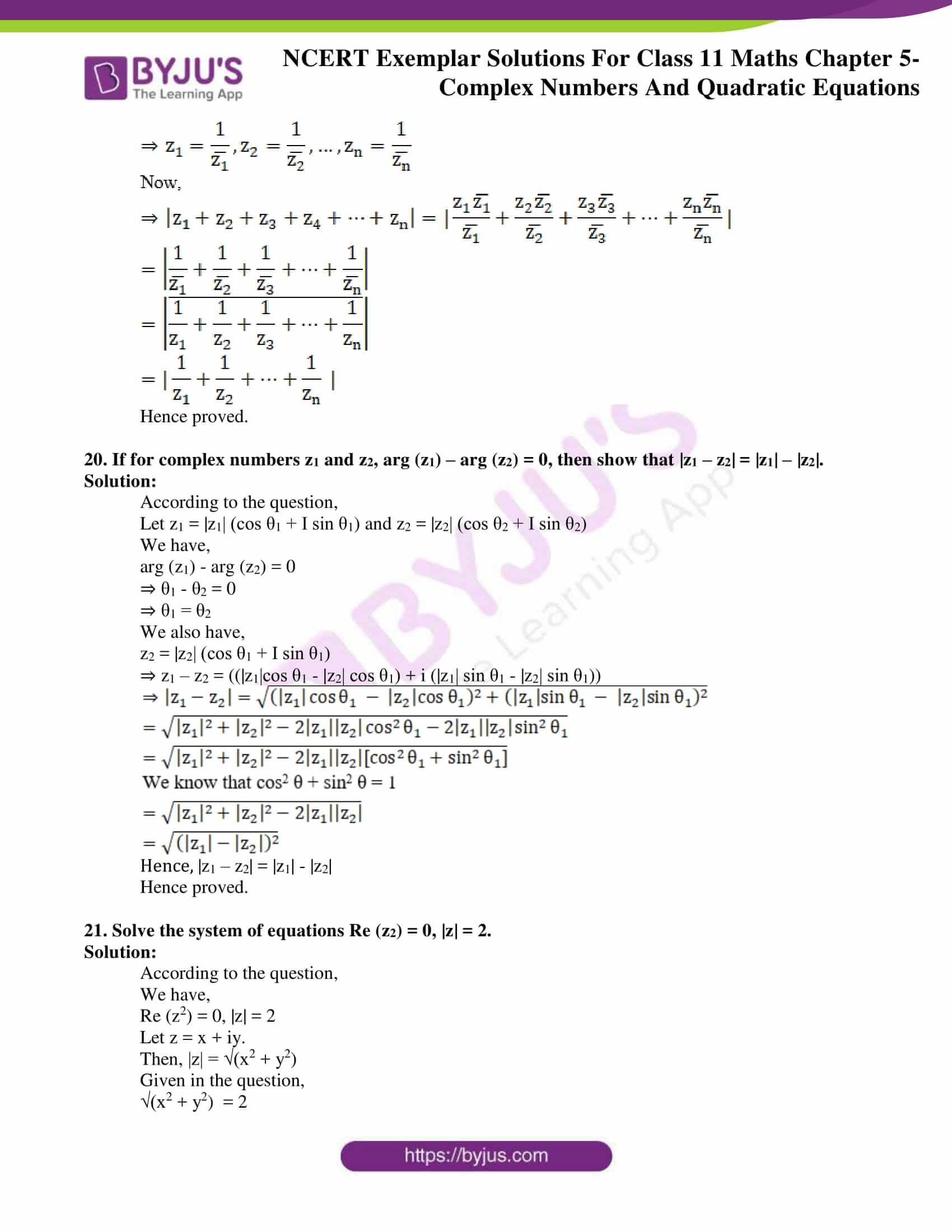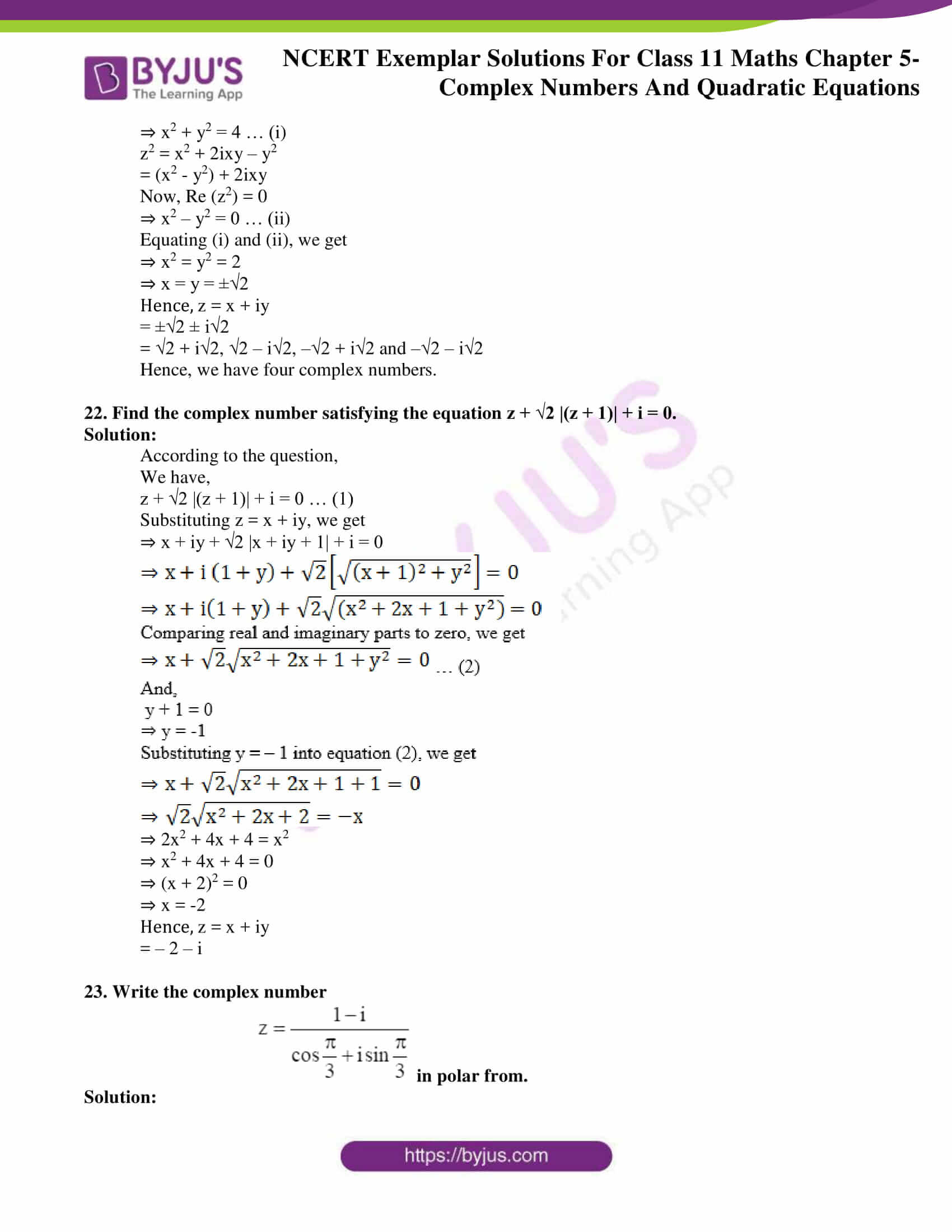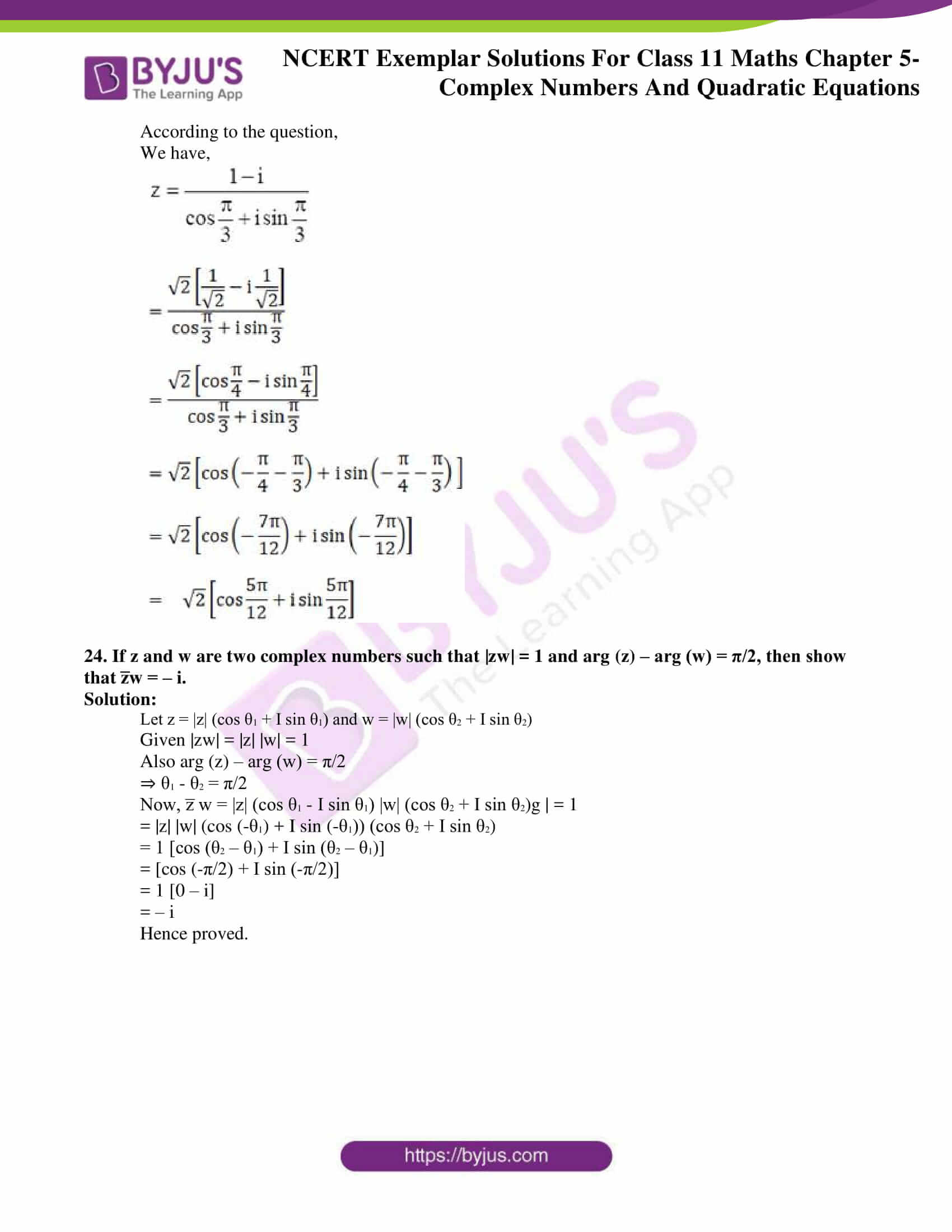### Access Answers to the NCERT Exemplar Class 11 Maths Chapter 5 – Complex Numbers and Quadratic Equations

Exercise Page No: 91

1. For a positive integer n, find the value of (1 – i)n
(1 – 1/i)n.

Solution:

According to the question,

We have,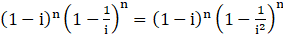= (1 – i)n (1 + i)n

= (1 – i2)n

= 2n

Therefore, (1 – i)n (1 – 1/i)n = 2n

2. Evaluate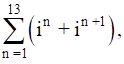where n ϵ N.

Solution:

According to the question,

We have,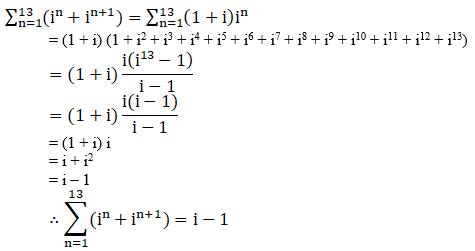3. If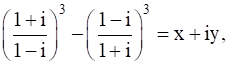, then find (x, y).

Solution:

According to the question,

We have,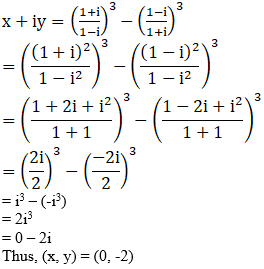= i3 – (-i3)

= 2i3

= 0 – 2i

Thus, (x, y) = (0, -2)

4. If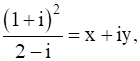, then find the value of x + y.

Solution:

According to the question,

We have,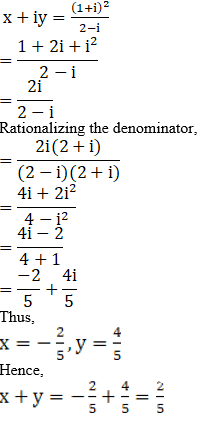5. If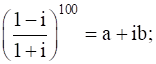then find (a, b).

Solution:

According to the question,

We have,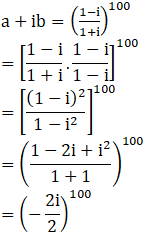= (i4)25

= 1

Hence, (a, b) = (1, 0)

6. If a = cos θ + i sin θ, find the value of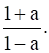Solution:

According to the question,

We have,

a = cos θ + i sin θ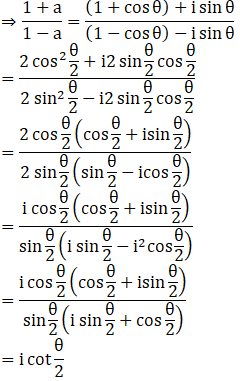7. If (1 + i)z = (1 – i) z̅, then show that z = i.

Solution:

According to the question,

We have,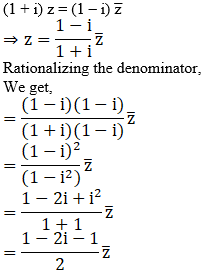= -iz̅

Hence proved.

8. If z = x + iy, then show that zz̅ + 2(z + z̅) + b = 0 where bϵR, representing z in the complex plane is a circle.

Solution:

According to the question,

We have,

z = x + iy

⇒ z̅ = x – iy

Now, we also have,

z z̅ + 2 (z + z̅) + b = 0

⇒ (x + iy) (x – iy) + 2 (x + iy + x – iy) + b = 0

⇒ x2 + y2 + 4x + b = 0

The equation obtained represents the equation of a circle.

9. If the real part of ( z̅ + 2)/ ( z̅ – 1) is 4, then show that the locus of the point representing z in the complex plane is a circle.

Solution:

According to the question,

Let z = x + iy

Now,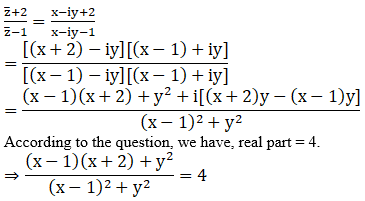⇒ x2 + x – 2 + y2 = 4 (x2 – 2x + 1 + y2)

⇒ 3x2 + 3y2 – 9x + 6 = 0

The equation obtained represents the equation of a circle.

Hence, locus of z is a circle.

10. Show that the complex number z, satisfying the condition arg ((z-1)/(z+1)) = π/4 lies on a circle.

Solution:

According to the question,

Let z = x + iy

arg ((z-1)/(z+1)) = π/4

⇒ arg (z – 1) – arg (z + 1) = π/4

⇒ arg (x + iy – 1) – arg (x + iy + 1) = π/4

⇒ arg (x – 1 + iy) – arg (x + 1 + iy) = π/4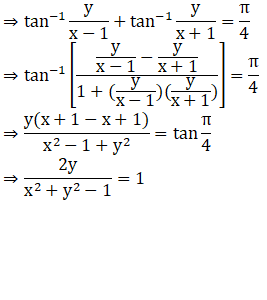⇒ x2 + y2 – 1 = 2y

⇒ x2 + y2 – 2y – 1 = 0

The equation obtained represents the equation of a circle.

11. Solve that equation |z| = z + 1 + 2i.

Solution:

According to the question,

We have,

|z| = z + 1 + 2i

Substituting z = x + iy, we get,

⇒ |x + iy| = x + iy + 1 + 2i

We know that,

|z| = √(x2 + y2)

√(x2 + y2) = (x + 1) + i(y + 2)

Comparing real and imaginary parts,

We get,

√(x2 + y2) = (x + 1)

And 0 = y + 2

⇒ y = -2

Substituting the value of y in √(x2 + y2) = (x + 1),

We get,

⇒ x2 + (-2)2 = (x + 1)2

⇒ x2 + 4 = x2 + 2x + 1

Hence, x = 3/2

Hence, z = x + iy

= 3/2 – 2i

12. If |z + 1| = z + 2 (1 + i), then find z.

Solution:

According to the question,

We have,

|z + 1| = z + 2 (1 + i)

Substituting z = x + iy, we get,

⇒ |x + iy + 1| = x + iy + 2 (1 + i)

We know,

|z| = √(x2 + y2)

√((x + 1)2 + y2) = (x + 2) + i(y + 1)

Comparing real and imaginary parts,

⇒ √((x + 1)2 + y2) = x + 2

And 0 = y + 2

⇒ y = -2

Substituting the value of y in √((x + 1)2 + y2) = x + 2,

⇒ (x + 1)2 + (-2)2 = (x + 2)2

⇒ x2 + 2x + 1 + 4 = x2 + 4x + 4

⇒ 2x = 1

Hence, x = ½

Hence, z = x + iy

= ½ – 2i

13. If arg (z – 1) = arg (z + 3i), then find x – 1 : y. where z = x + iy

Solution:

According to the question,

Let z = x + iy

Given that,

arg (z – 1) = arg (z + 3i)

⇒ arg (x + iy – 1) = arg (x + iy + 3i)

⇒ arg (x – 1 + iy) = arg (x + I (y) = π/4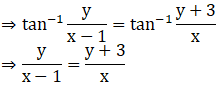⇒ xy = xy – y + 3x – 3

⇒ 3x – 3 = y

⇒ (x – 1)/y = 1/3

Hence, (x – 1): y = 1: 3

14. Show that |(z – 2) / (z – 3)| = 2 represents a circle. Find its centre and radius.

Solution:

According to the question,

We have,

|(z – 2) / (z – 3)| = 2

Substituting z = x + iy, we get

⇒ |(x + iy – 2) / (x + iy – 3)| = 2

⇒ |x – 2 + iy| = 2 |x – 3 + iy|

⇒ √((x – 2)2 + y2) = 2√((x – 3)2 + y2)

⇒ x2 – 4x + 4 + y2 = 4 (x2 – 6x + 9 + y2)

⇒ 3x2 + 3y2 – 20x + 32 = 0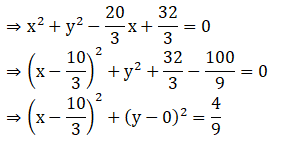Therefore, centre of circle is (10/3, 0) and radius is 4/9 or 2/3.

15. If (z – 1)/(z + 1) is a purely imaginary number (z ≠ –1), then find the value of |z|.

Solution:

According to the question,

Let z = x + iy

Now,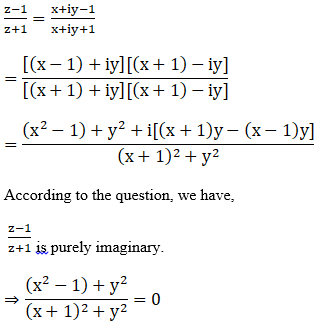⇒ x2 – 1 + y2 = 0

⇒ x2 + y2 = 1

⇒ √(x2 + y2) = 1

Hence, |z| = 1

16. z1 and z2 are two complex numbers such that |z1| = |z2| and arg (z1) + arg (z2) = π, then show that z1 = – z̅2.

Solution:

According to the question,

Let z1 = |z1| (cos θ1 + I sin θ1) and z2 = |z2| (cos θ2 + I sin θ2)

Given that |z1| = |z2|

And arg (z1) + arg (z2) = π

⇒ θ1 + θ2 = π

⇒ θ1 = π – θ2

Now, z1 = |z2| (cos (π – θ2) + I sin (π – θ2))

⇒ z1 = |z2| (-cos θ2 + I sin θ2)

⇒ z1 = -|z2| (cos θ2 – I sin θ2)

⇒ z1 = – [|z2| (cos θ2 – I sin θ2)]

Hence, z1 = -z̅2

Hence proved.

17. If |z1| = 1 (z1 ≠ –1) and z2 = (z1 – 1) / (z + 1), then show that the real part of z2 is zero.

Solution:

According to the question,

Let z1 = x + iy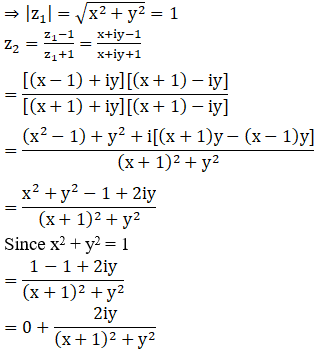Therefore, the real part of z2 is zero.

18. If z1, z2 and z3, z4 are two pairs of conjugate complex numbers, then find arg(z1/z4) + arg(z2/z3).

Solution:

According to the question,

We have,

z1 and z2 are conjugate complex numbers.

The negative side of the real axis

= r1 (cos θ1 – i sin θ1)

= r1 [cos (-θ1) + I sin (-θ1)]

Similarly, z3 = r2 (cos θ2 – i sin θ2)

⇒ z4 = r2 [cos (-θ2) + I sin (-θ2)]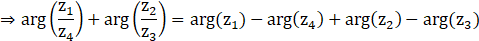= θ1 – (-θ2) + (-θ1) – θ2

= θ1 + θ2 – θ1 – θ2

= 0

⇒ arg(z1/z4) + arg(z2/z3) = 0

19. If |z1| = |z2| = ….. = |zn| = 1, then show that |z1 + z2 + z3 + …. + zn| = | 1/z1 + 1/z2 + 1/z3 + … + 1/zn|

Solution:

According to the question,

We have,

|z1| = |z2| = … = |zn| = 1

⇒ |z1|2 = |z2|2 = … = |zn|2 = 1

⇒ z1 z̅ 1= z2 z̅ 2= z3 z̅ 3= … = zn z̅ n= 1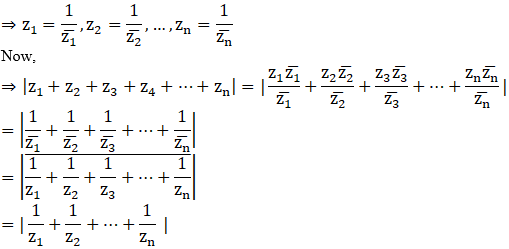Hence proved.

20. If for complex numbers z1 and z2, arg (z1) – arg (z2) = 0, then show that |z1 – z2| = |z1| – |z2|.

Solution:

According to the question,

Let z1 = |z1| (cos θ1 + I sin θ1) and z2 = |z2| (cos θ2 + I sin θ2)

We have,

arg (z1) – arg (z2) = 0

⇒ θ1 – θ2 = 0

⇒ θ1 = θ2

We also have,

z2 = |z2| (cos θ1 + I sin θ1)

⇒ z1 – z2 = ((|z1|cos θ1 – |z2| cos θ1) + i (|z1| sin θ1 – |z2| sin θ1))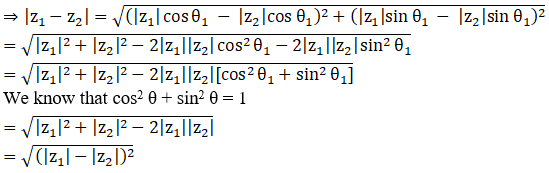Hence, |z1 – z2| = |z1| – |z2|

Hence proved.

21. Solve the system of equations Re (z2) = 0, |z| = 2.

Solution:

According to the question,

We have,

Re (z2) = 0, |z| = 2

Let z = x + iy.

Then, |z| = √(x2 + y2)

Given in the question,

√(x2 + y2)  = 2

⇒ x2 + y2 = 4 … (i)

z2 = x2 + 2ixy – y2

= (x2 – y2) + 2ixy

Now, Re (z2) = 0

⇒ x2 – y2 = 0 … (ii)

Equating (i) and (ii), we get

⇒ x2 = y2 = 2

⇒ x = y = ±√2

Hence, z = x + iy

= ±√2 ± i√2

= √2 + i√2, √2 – i√2, –√2 + i√2 and –√2 – i√2

Hence, we have four complex numbers.

22. Find the complex number satisfying the equation z + √2 |(z + 1)| + i = 0.

Solution:

According to the question,

We have,

z + √2 |(z + 1)| + i = 0 … (1)

Substituting z = x + iy, we get

⇒ x + iy + √2 |x + iy + 1| + i = 0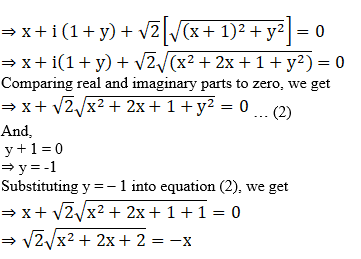⇒ 2x2 + 4x + 4 = x2

⇒ x2 + 4x + 4 = 0

⇒ (x + 2)2 = 0

⇒ x = -2

Hence, z = x + iy

= – 2 – i

23. Write the complex number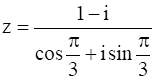in polar from.

Solution:

According to the question,

We have,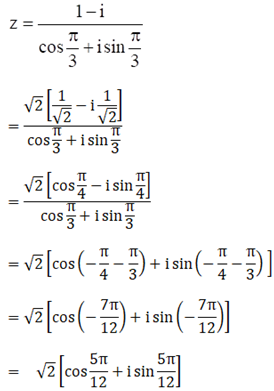24. If z and w are two complex numbers such that |zw| = 1 and arg (z) – arg (w) = π/2, then show that z̅w = – i.

Solution:

Let z = |z| (cos θ1 + I sin θ1) and w = |w| (cos θ2 + I sin θ2)

Given |zw| = |z| |w| = 1

Also arg (z) – arg (w) = π/2

⇒ θ1 – θ2 = π/2

Now, z̅ w = |z| (cos θ1 – I sin θ1) |w| (cos θ2 + I sin θ2)g | = 1

= |z| |w| (cos (-θ1) + I sin (-θ1)) (cos θ2 + I sin θ2)

= 1 [cos (θ2 – θ1) + I sin (θ2 – θ1)]

= [cos (-π/2) + I sin (-π/2)]

= 1 [0 – i]

= – i

Hence proved